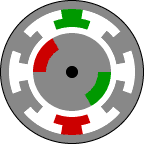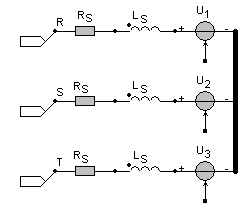• Introduction
• What is in this manual
• What is Caspoc
• User interface
• Introduction
• Starting
• Simulation
• Editing
• Viewing and printing
• Getting Started
• Basic editing
• Simulation in the time domain
• Basic User Interface Topics
• Editing
• Simulation
• Viewing
• Library
• Reports
• Project management
• Circuit and Block Diagram Components
• Introduction
• Cscript and user defined functions
• Component parameters
• Modeling Topics
• Introduction
• Power Electronics
• Semiconductors
• Electrical Machines
• Electrical drives
• Power Systems
• Mechanical Systems
• Thermal Systems
• Magnetic Circuits
• Green Energy
• Coupling to FEM
• Experimenter
• Analog hardware description language
• Embedded C code Export
• Coupling to Spice
• Small Signal Analysis
• Matlab coupling
• Tips and tricks
• Appendices

## Brushless DC MotorsClick to Close this View Brushless DC motors are refered to by many aliases: brushless permanent magnet, permanent magnet ac motors, permanent magnet synchronous motors ect. The confusion arises because a brushless dc motor does not directly operate off a dc voltage source. However, as we shall see, the basic principle of operation is similar to a dc motor.

A brushless dc motor has a rotor with permanent magnets and a stator with windings. It is essentially a dc motor turned inside out. The brushes and commutator have been eliminated and the windings are connected to the control electronics. The control electronics replace the function of the commutator and energize the proper winding.

As shown in the animation the windings are energized in a pattern which rotates around the stator. The energized stator winding leads the rotor magnet, and switches just as the rotor aligns with the stator.

There are no sparks, which is one advantage of the bldc motor. The brushes of a dc motor have several limitations; brush life, brush residue, maximum speed, and electrical noise. BLDC motors are potentially cleaner, faster, more efficient, less noisy and more reliable. However, BLDC motors require electronic control.

The basic block BLDCM from the block diagram models the Brushless types of DC machines. The output equals the electric torque produced by the machine. The inputs are the three phase currents, the angular speed of the rotor and the machine constant for the calculation of the torque and the produced EMF.

u1:=K*sin(angle);u2:=K*sin(angle-(2*PI/3));u3:=K*sin(angle-(4*PI/3));if(p1=0)then  begin  if(u1 < 0) then u1:=K else u1:=-K;  if(u2 < 0) then u2:=K else u2:=-K;  if(u3 < 0) then u3:=K else u3:=-K;  end;T=u1*i1+u2*i2+u3*i3

Inside the basic BLDCM block from the block diagram the type of the machine can be defined.

p1=0 Brushless DC machine
p1=1 Brushless AC machine

Use an INT_VAR block to get the EMF voltages and position of the rotor:

 INT_VAR Output p1=1 u1 p1=2 u2 p1=3 u3 p1=4 Position of the rotor (in Radians) p1=5 Rotor position polar x-coordinate p1=6 Rotor position polar y-coordinate

Equivalent circuit model

The library blocks for the Brushless DC machines have electrical and mechanical connections. The electrical part of the BLDCM is modeled by a series connection of the stator inductance, resistance and the above mentioned EMF.The connections R, S and T are the electrical terminals. The stator current is depends on the produced EMF:

us=Rs · is+Lsdis/dt+uEMF)

where uEMFr*ui

The torque produced by the machine equals:

Te=u1 · i1+u2 · i2+u3 · i3

Mechanical part:

The mechanical shaft is modeled by a rigid shaft model. The inertia J [kgm2]of the shaft can be modeled in most of the BLDCM library blocks. Most machine models have internal parameters for modeling the inertia and friction of the shaft. The Brushless DC machine can either run without load or can be connected to a mechanical rotational model. Use the models from components/circuit/rotational and from the various mechanical libraries for modeling the mechanical model.